Practice the problems of Math in Focus Grade 4 Workbook Answer Key Mid Year Review to score better marks in the exam.

Multiple Choice
Fill in the circle next to the correct answer.
Question 1.
13 thousands + 4 tens + 8 ones in standard form is ___ (Lesson 1.1)
(A) 1,348
(B) 10,348
(C) 13,048
(D) 13,480
13 thousands + 4 tens + 8 ones in standard form = 13048.
(C) 13,048.

Explanation:
13 thousands + 4 tens + 8 ones in standard form = 13000 + 40 + 8
= 13040 + 8
= 13048.

Question 2.
In the number 83.415 the value of the digit 3 is ____. (Lesson 1.1)
(A) 30
(B) 300
(C) 3,000
(D) 30,000
In the number 83.415, the value of the digit 3 is 3000.
(C) 3,000

Explanation:
In the number 83.415,
8 tens 3 ones 4 tenths 1 hundredths 5 thousandths.
the value of the digit 3 is 3000.

Question 3.
1.000 more than 37,568 is ____ (Lesson 1.2)
(A) 36,568
(B) 37,468
(C) 37,668
(D) 38,568
1.000 more than 37,568 = 38,568.
(D) 38,568.

Explanation:
1.000 more than 37,568 = 37,568 + 1000 = 38,568.

Question 4.
Estimate 681 – 307 by rounding to the nearest 100. (Lesson 2.1)
(A) 300
(B) 370
(C) 374
(D) 400
Estimate 681 – 307 by rounding to the nearest 100 = 400.
(D) 400

Explanation:
Estimate 681 – 307 by rounding to the nearest 100:
681 nearest hundred = 700.
307 nearest hundred = 300.
Difference 681 – 307 = 700 – 300 = 400.

Question 5.
Which is the greatest common factor of 27 and 45? (Lesson 2.2)
(A) 1
(B) 3
(C) 9
(D) 45
Greatest common factor of 27 and 45 = 9.
(C) 9.

Explanation:
Greatest common factor of 27 and 45 = 9.
3 × 9 = 27.
5 × 9 = 45.

Question 6.
Which pair of numbers has both a prime and a composite number? (Lesson 2.2)
(A) 4 and 7
(B) 3 and 13
(C) 14 and 28
(D) 6 and 8
Pair of numbers has both a prime and a composite number: 3 and 13.
(B) 3 and 13.

Explanation:
A prime number is a whole number greater than 1. It has exactly two factors, that is, 1 and the number itself.
A composite number is a positive integer that can be formed by multiplying two smaller positive integers. Equivalently, it is a positive integer that has at least one divisor other than 1 and itself.
Pair of numbers has both a prime and a composite number:
A. 4 and 7 = (2 × 2) and (1 × 7)
B. 3 and 13 = (1 × 3) and (1 × 13)
C. 14 and 18 = [(2 × 7) (1 × 7)] and  [(2 × 9) (3 × 3)]
D. 6 and 8 = [(2 × 3) (1 × 6)] and [(2 × 4) (1 × 8)]

Question 7.
What is the sum of the first two multiples of 6? (Lesson 2.3)
(A) 3
(B) 6
(C) 12
(D) 18
Sum of the first two multiples of 6: 18.
(D) 18.

Explanation:
Sum of the first two multiples of 6:
6 × 1 = 6.
2 × 6 = 12.
Sum = 6 + 12 = 18.

Question 8.
Mr. Finch exercises at the gym every two days. Mr. Chavez exercises at the gym every five days. When will they meet next if they first met on January 5? (Lesson 2.3)
(A) January 7
(B) January 10
(D) January 25
Next day when they meet = (2 × 5) = January 10.
(B) January 10.

Explanation:
Number of days Mr. Finch exercises at the gym = every 2.
Number of days Mr. Chavez  exercises at the gym = every 5.
Next day when they meet = (2 × 5) = January 10.

Question 9.
Divide 5,61 3 by 7. The remainder is ____ (Lesson 3.4)
(A) 1
(B) 6
(C) 18
(D) 81
Divide 5,61 3 by 7. The remainder is 6.
(B) 6.

Explanation:
5613 ÷ 7 = 801 $$\frac{6}{7}$$

Question 10.
After using 35 jars to store 14 marbles each, Ali has 3 marbles left. How many marbles did he have at first? (Lesson 3.5)
(A) 52
(B) 178
(C) 490
(D) 493
Number of marbles Alia has first = 493.
(D) 493.

Explanation:
Number of jars store using = 35.
Number of marbles each jar has = 14.
Number of marbles left = 3.
Number of marbles Alia has first = (Number of jars store using × Number of marbles each jar has) + Number of marbles left
= (35 × 14) + 3
= 490 + 3
= 493.

Question 11.
The table shows the medals different teams won at a competition. (Lesson 4.2)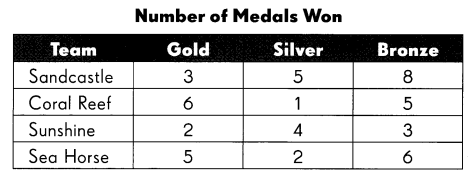At which intersection was one medal won?
(A) Sandcastle and Gold
(B) Coral Reef and Silver
(C) Sunshine and Bronze
(D) Seahorse and Silver
Intersection one medal won = Coral Reef and Silver.
(B) Coral Reef and Silver.

Explanation:
Intersection one medal won = Coral Reef and Silver.Question 12.
Find the mode. (Lesson 5.2)
31 lb 36 lb 21 lb 40 lb 38 lb 40 lb
(A) 31 lb
(B) 36 lb
(C) 37 lb
(D) 40 lb
Mode of 31 lb 36 lb 21 lb 40 lb 38 lb 40 lb = 40lb.
(D) 40 lb.

Explanation:
Mode:
31 lb 36 lb 21 lb 40 lb 38 lb 40 lb
31 = 1.
36 = 1.
21 = 1.
40 = 2.
38 = 1.

Question 13.
Jim ordered cons of fruit cocktail for his diner for 6 months. (Lesson 5.3)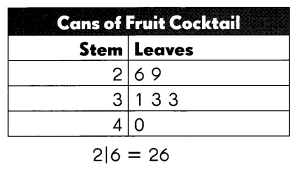What is the median number of cans he ordered?
(A) 29 cans
(B) 32 cans
(C) 33 cans
(D) 40 cans
Median number of cans he ordered = 33 cans.

Explanation:
Median is the middle number in a sorted list of numbers. To determine the median value in a sequence of numbers, the numbers must first be sorted, or arranged, in value order from lowest to highest or highest to lowest.
0 1 3 3 6 9
Median number of cans he ordered = 33 cans.

Question 15.
Stacy draws one of these number cards from a bag.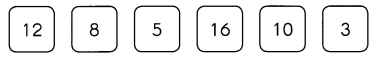What is the probability that she draws a number less than 10? (Lesson 5.5)
(A) $$\frac{1}{2}$$
(B) $$\frac{1}{3}$$
(C) $$\frac{2}{3}$$
(D) $$\frac{1}{6}$$
Probability that she draws a number less than 10 = $$\frac{1}{6}$$
(D) $$\frac{1}{6}$$

Explanation:
Probability is simply how likely something is to happen. Whenever we’re unsure about the outcome of an event, we can talk about the probabilities of certain outcomes—how likely they are. The analysis of events governed by probability is called statistics.Probability that she draws a number less than 10 = card less than 10 ÷ total number of cards.
= 1 ÷ 6 or $$\frac{1}{6}$$.

Question 16.
Which two fractions have a sum of $$\frac{9}{10}$$? (Lesson 6.1)
(A) $$\frac{1}{2}$$ and $$\frac{4}{10}$$
(B) $$\frac{1}{2}$$ and $$\frac{1}{10}$$
(C) $$\frac{2}{5}$$ and $$\frac{1}{10}$$
(D) $$\frac{3}{4}$$ and $$\frac{6}{6}$$
Two fractions have a sum of $$\frac{9}{10}$$ = (A)
(A) $$\frac{1}{2}$$ and $$\frac{4}{10}$$

Explanation:
(A) $$\frac{1}{2}$$ and $$\frac{4}{10}$$
Sum = 0.5 + 0.4 = 0.9.
(B) $$\frac{1}{2}$$ and $$\frac{1}{10}$$
Sum = 0.5 + 0.1 = 0.6.
(C) $$\frac{2}{5}$$ and $$\frac{1}{10}$$
Sum = 0.4 + 0.1 = 0.5.
(D) $$\frac{3}{4}$$ and $$\frac{6}{6}$$
Sum = 0.75 + 1 = 1.75.

Question 17.
Which mixed number is represented by A on the number line? (Lesson 6.3)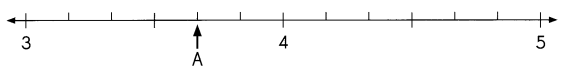(A) 3$$\frac{4}{5}$$
(B) 3$$\frac{2}{3}$$
(C) 4$$\frac{1}{3}$$
(D) 4$$\frac{2}{3}$$
A = 3.8.
(A) 3$$\frac{4}{5}$$.

Explanation:
(A) 3$$\frac{4}{5}$$ = (15 + 4) ÷ 5 = $$\frac{19}{5}$$ = 3.8.
(B) 3$$\frac{2}{3}$$ = (9 + 2) ÷ 3 = $$\frac{11}{3}$$ = 3.67.
(C) 4$$\frac{1}{3}$$ = (12 + 1) ÷ 3 = $$\frac{13}{3}$$ = 4.33.
(D) 4$$\frac{2}{3}$$ = (12 + 2) ÷ 3 = $$\frac{14}{3}$$ = 4.67.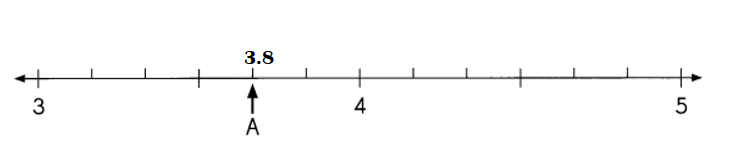Question 18.
How many fifths are in 2$$\frac{3}{5}$$ ? (Lesson 6.4)
(A) 10
(B) 11
(C) 13
(D) 23
13 fifths are in 2$$\frac{3}{5}$$.
(C) 13.

Explanation:
2$$\frac{3}{5}$$ = (10 + 3) ÷ 5 = $$\frac{13}{5}$$

Question 19.
Express $$\frac{14}{6}$$ as a mixed number in simplest form. (Lesson 65)
(A) 1$$\frac{4}{6}$$
(B) 1$$\frac{2}{3}$$
(C) 2$$\frac{2}{6}$$
(D) 2$$\frac{1}{3}$$
Simplest form of $$\frac{14}{6}$$ = 2$$\frac{2}{6}$$
(C) 2$$\frac{2}{6}$$

Explanation:
Simplest form of $$\frac{14}{6}$$ = 2$$\frac{2}{6}$$

Question 20.
Ms. Lee cut a piece of yarn into different fractional parts:
$$\frac{1}{12}$$, $$\frac{1}{4}$$ and $$\frac{5}{12}$$. What fraction of the yarn is left? (Lesson 6.7)
(A) $$\frac{1}{4}$$
(B) $$\frac{5}{12}$$
(C) $$\frac{8}{12}$$
(D) $$\frac{3}{4}$$
Fraction of the yarn is left = $$\frac{1}{4}$$.
(A) $$\frac{1}{4}$$

Explanation:
$$\frac{1}{12}$$, $$\frac{1}{4}$$ and $$\frac{5}{12}$$.
Fraction of the yarn is left = 1 – ($$\frac{1}{12}$$ +  $$\frac{1}{4}$$ + $$\frac{5}{12}$$)
= 1 – (0.08 + 0.25 + 0.42)
= 1 – (0.33 + 0.42)
= 1 – 0.75
= 0.2 or$$\frac{1}{4}$$

Question 21.
Write forty thousand, sixteen in expanded form. (Lesson 1.1)
Expanded form of forty thousand, sixteen = 40,016.

Explanation:
Expanded form of forty thousand, sixteen = 40,000 + 16 = 40,016.

Question 22.
Arrange the numbers in order from least to greatest. (Lesson 1.2)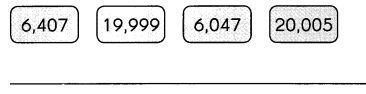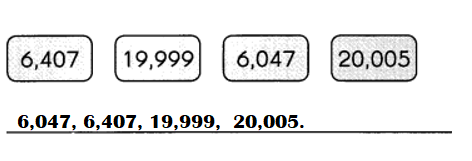Explanation:
Numbers in order from least to greatest :
6,047, 6,407, 19,999, 20,005.

Question 23.
Estimate the quotient of 713 ÷ 9. (Lesson 2.1)
____
Quotient of 713 ÷ 9 = 79.

Explanation:
Quotient of 713 ÷ 9 = 79$$\frac{2}{9}$$.

Question 24.
The table shows the number of people who visited the space ride at a theme park. Complete the table. (Lesson 4.2)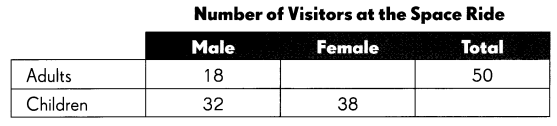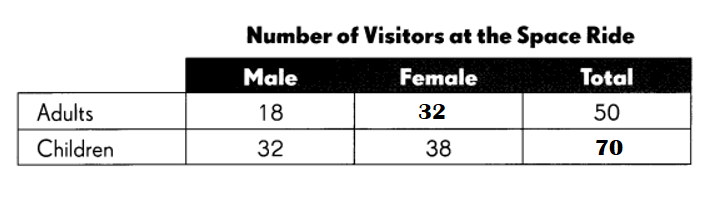Explanation:
Number of male who visited the space ride at a theme park = 18.
Total adults who visited the space ride at a theme park = 50.
Number of female who visited the space ride at a theme park = Total adults who visited the space ride at a theme park – Number of male who visited the space ride at a theme park
= 50 – 18
= 32.
Number of male children who visited the space ride at a theme park = 32.
Number of female children who visited the space ride at a theme park = 38.
Total number of children who visited the space ride at a theme park = Number of male children who visited the space ride at a theme park + Number of female children who visited the space ride at a theme park
= 32 + 38
= 70.

Use the table to answer Exercises 25 and 26.
Question 25.
How many people visited the space ride? (Lesson 4.2) ____
Total number of people who visited the space ride at a theme park = 120.

Explanation:
Total adults who visited the space ride at a theme park = 50.
Total number of children who visited the space ride at a theme park = 70.
Total number of people who visited the space ride at a theme park = Total adults who visited the space ride at a theme park + Total number of children who visited the space ride at a theme park
= 50 + 70
= 120.

Question 26.
What fraction of the people who visited the space ride were children? (Lesson 6.7) _____
Fraction of the people who visited the space ride were children = 7 ÷ 12 or $$\frac{7}{12}$$.

Explanation:
Total number of children who visited the space ride at a theme park = 70.
Total number of people who visited the space ride at a theme park = 120.
Fraction of the people who visited the space ride were children = Total number of children who visited the space ride at a theme park ÷ Total number of people who visited the space ride at a theme park
= 70 ÷ 120
= 7 ÷ 12 or $$\frac{7}{12}$$.

The line graph shows the number of visitors at a museum during the course of a day. (Lesson 4.3)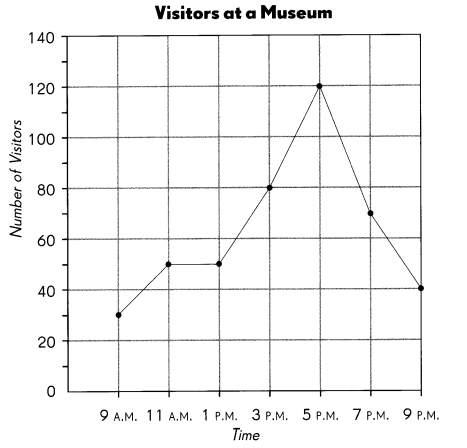Question 27.
By how much did the visitor population increase from 1:00 P.M. to 3:00 P.M.? ______________
35 visitor population increased from 1:00 P.M. to 3:00 P.M.

Explanation:
Visitor Population at 1:00 P.M = 45.
Visitor Population at 3:00 P.M = 80.
Difference:
Visitor Population at 3:00 P.M – Visitor Population at 1:00 P.M
= 80 – 45
= 35.

Question 28.
During which interval did the visitor population decrease the most? ___
5:00 P.M to 7:00 P.M interval did the visitor population decrease the most of 55.

Explanation:
Visitor Population at 9:00 A.M = 25.
Visitor Population at 11:00 A.M = 45.
Visitor Population at 1:00 P.M = 45.
Visitor Population at 3:00 P.M = 80.
Visitor Population at 5:00 P.M = 120.
Visitor Population at 7:00 P.M = 65.
Visitor Population at 9:00 P.M = 40.
5:00 P.M to 7:00 P.M interval
= Visitor Population at 5:00 P.M – Visitor Population at 7:00 P.M
= 120 – 65
= 55.

Question 29.
During which interval did the same number of visitors arrive and depart?
11:00 A.M interval did the same number of visitors arrive and depart.

Explanation:
Visitor Population at 11:00 A.M = 45.
Visitor Population at 1:00 P.M = 45.

Use the line plot to solve Exercises 30 and 31. (Lesson 5.2)
The line plot shows the number of siblings each student in John’s class has.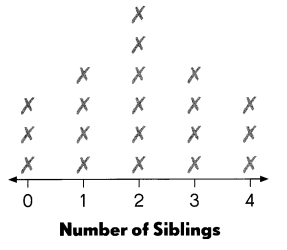Question 30.
Find the median of the set of data. ____
Median of the set of data = 2.

Explanation:
Number of siblings = 0 1 2 3 4
Median of the set of data = 2.

Question 31.
Find the mode of the set of data. ____
Mode of the set of data = 2.

Explanation:
The mode is the value that appears most often in a set of data values. If X is a discrete random variable, the mode is the value x (i.e, X = x) at which the probability mass function takes its maximum value. In other words, it is the value that is most likely to be sampled.
Number of siblings in 2 are more.

Use the stem-and-leaf plot to solve Exercises 32 and 33. (Lesson 5.3)
The stem-and-leaf plot shows the number of orchids produced by 10 greenhouse plants in one month.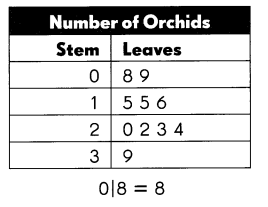Question 32.
The median of the set of data is ___
Median of the set of data = 5.

Explanation:
0 2 3 4 5 5 6 8 9 9
Median of the set of data = (5 + 5 ) ÷ 2
= 10 ÷ 2
= 5.

Question 33.
The outlier of the set of data is ___.
The outlier of the set of data is 6.

Explanation:
Outliers are data points that don’t fit the pattern of rest of the numbers. They are the extremely high or extremely low values in the data set. A simple way to find an outlier is to examine the numbers in the data set.
8  9  5   5  6  0  2  3  4  9

Look at the spinner. Write more likely, less likely, equally likely, certain, or impossible. Explain your answer. (Lesson 5.4)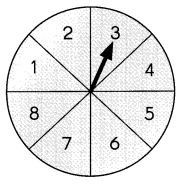Question 34.
The spinner is ___ to land on an odd number or an even number.
Reason: ____
The spinner is certain to land on an odd number.

Explanation:
The spinner is certain to land on an odd number because spinner hand is on 3 (odd number) not even number.

The bar graph shows the color of the horses at a horse show.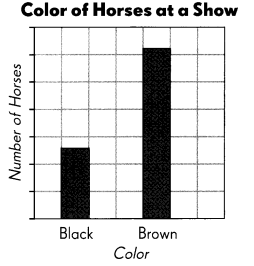Question 35.
Which set is more likely to be the one shown in the bar graph? (Lesson 5.4)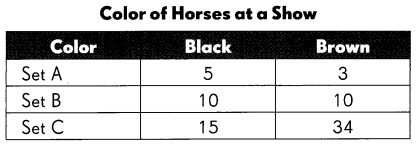Set C is more likely to be the one shown in the bar graph.

Explanation:
Set C is more likely to be the one shown in the bar graph because black is more likely to be half of the brown horse.

Question 36.
A bag has 5 red beads, 8 green beads, and 4 yellow beads. What is the probability of drawing a yellow bead from the bag? (Lesson 5.5)
______________
Probability of drawing a yellow bead from the bag = 4 ÷ 17 or $$\frac{4}{17}$$.

Explanation:
Number of yellow beads = 4.
= 5 + 8 + 4
= 13 + 4
= 17.
Probability of drawing a yellow bead from the bag = Number of yellow beads ÷ Total number of beads
= 4 ÷ 17 or $$\frac{4}{17}$$.

Question 37.
Find the sum of $$\frac{1}{6}$$, $$\frac{1}{6}$$, and $$\frac{2}{3}$$. (Lesson 6.1)
Sum of $$\frac{1}{6}$$, $$\frac{1}{6}$$, and $$\frac{2}{3}$$ = 1.

Explanation:
Sum = $$\frac{1}{6}$$ +  $$\frac{1}{6}$$ + $$\frac{2}{3}$$
=[1 + 1 + (2 × 2) ] ÷ 6
= (2 + 4) ÷ 6
= 6 ÷ 6 or $$\frac{6}{6}$$
= 1.

Question 38.
What is 1$$\frac{1}{4}$$ – $$\frac{5}{8}$$? (Lesson 6.6)
1$$\frac{1}{4}$$ – $$\frac{5}{8}$$ = 5 ÷ 8 or $$\frac{5}{8}$$.

Explanation:
Difference:
1$$\frac{1}{4}$$ – $$\frac{5}{8}$$
= (4 + 1) ÷ 4 – $$\frac{5}{8}$$
= $$\frac{5}{4}$$ – $$\frac{5}{8}$$
= $$\frac{10}{8}$$ – $$\frac{5}{8}$$
= (10 – 5) ÷ 8
= 5 ÷ 8 or $$\frac{5}{8}$$.

Question 39.
A container and 6 lemons have a total weight of $$\frac{4}{5}$$ pound. Two lemons have a total weight of pound. Find the weight of the container if all the lemons have the same weight. (Lesson 6.8)

Explanation:

Extended Response
Question 40.
A clinic needs 1,350 chairs for a charity event. Three stores donate chairs. Store A donates 216 chairs, Store B donates 420 chairs, and Store C donates 376 chairs. Does the clinic have enough chairs? Decide if you need to find an estimate or an exact answer. (Lesson 2.1)
338 more chairs are needed.

Explanation:
Number of chairs Store A donates = 216.
Number of chairs Store B donates = 420.
Number of chairs Store C donates = 376.
Total number of chairs a clinic needs for a charity event = 1,350.
Number of chairs all Stores donated = Number of chairs Store A donates + Number of chairs Store B donates + Number of chairs Store C donates
= 216 + 420 + 376
= 636 + 376
= 1012.
Difference:
Total number of chairs a clinic needs for a charity event – Number of chairs all Stores donated
= 1,350 – 1,012
= 338.

Question 41.
Barrie had some stamps. He gave $$\frac{1}{8}$$ of them to Tom. If he gave 15 stamps to Tom, how many stamps did he have at first? (Lesson 6.8)
Number of stamps he have first = 120.

Explanation:
Fraction of stamps he gave to Tom = $$\frac{1}{8}$$
Number of stamps if he gave to him = 15.
Number of stamps he have first = Number of stamps if he gave to him ÷ Fraction of stamps he gave to Tom
= 15 ÷ $$\frac{1}{8}$$
= 15 × 8
= 120.

Question 42.
Mr. Marchez ordered 7 books through a website. The total mass of the books was 3,458 grams. The masses of each book were 360 g 410 g 280 g 150 g 550 g ? ?
The masses of the remaining 2 books were not given. (Lesson 5.6)

a. Find the mean mass of the books.
Mean mass of the books = 494 grams.

Explanation:
The mean is the average of the numbers
The masses of each book were 360 g 410 g 280 g 150 g 550 g
Total mass of all books = 3,458 grams.
Number of books = 7.
Mean mass of the books = Total mass of all books ÷ Number of books
= 3,458 ÷ 7
= 494 grams.

b. Find the mean mass of the 2 remaining books.
Mass of the remaining 2 books = 1,708.

Explanation:
Mass of the remaining 2 books =
(Total mass of all books – Mass of 5 books)
= 3,458  – (360 + 410 + 280 + 150 + 550)
= 3,458  – (770 + 280 + 150 + 550)
= 3,458  – (1,050 + 150 + 550)
= 3,458  – (1,200 + 550)
= 3,458  – 1,750
= 1,708.

c. The range of the masses is 710 grams, and the lightest mass is given above. What is the mass of the heaviest book?
Mass of the heaviest book = 550 grams.

Explanation:
Range of the masses = 710 grams.
The masses of each book were 360 g 410 g 280 g 150 g 550 g.
=> lightest mass = 150grams.
Mass of the heaviest book = 550 grams.

Question 43.
A factory packages 4,250 boxes of cereal. The number of oat cereal boxes is 715 more than the number of wheat cereal boxes. The number of fruit cereal boxes is 5 times the number of wheat cereal boxes. How many fruit cereal boxes does the factory package? (Lesson 3.5)
Number of fruits cereal boxes = 2,525.

Explanation:
Number of boxes of cereal a factory packages = 4,250.
The number of oat cereal boxes is 715 more than the number of wheat cereal boxes.
Let the wheat cereal boxes be X.
=> Number of oat cereal boxes = 715 + X.
The number of fruit cereal boxes is 5 times the number of wheat cereal boxes.
=> Number of fruits cereal boxes = 5 × X = 5X.
Number of boxes of cereal a factory packages = Number of oat cereal boxes + Number of fruits cereal boxes + Number of wheat cereal boxes
=> 4250 =  (715 + X) + 5X + X
=> 4250 = 715 + X + 5X + X
=> 4250 = 715 + 2X + 5X
=> 4250 = 715 + 7X
=> 4250 – 715 = 7X
=> 3535 = 7X
=> 3535 ÷ 7 = X
=> 505 = X.
=> Number of wheat cereal boxes  = 505.
Number of oats cereal boxes = 715 + X = 715 + 505 = 1,220.
Number of fruits cereal boxes = 5X = 5 × 505 = 2,525.

Question 44.
Three people guess the number of cherries in a bag, rounded to the nearest 10. Alex guesses 80 cherries, Jess guesses 60 cherries, and Nia guesses 70 cherries. The actual number is a multiple of 7. The difference of the digits of the number is 9. Who guessed correctly? (Lesson 2.1 and 2.2)